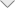### Radar Cross Section Benchmark for Simple Shapes

Introduction

This application demonstrates Radar Cross Section (RCS) calculation for simple shapes including the NASA almond, ogive, double-ogive, cone-sphere and cone-sphere with gap. Simulation data is compared with published measurements data from A. C. Woo, H. T. G. Wang, and M. J. Schuh, “Benchmark Radar Targets for the Validation of Computational Electromagnetics Programs,” IEEE Antennas and Propagation Magazine, vol. 35, no. 1, February 1993, pp. 84 – 89.

NASA Metallic Almond

The NASA almond geometry and analytical expressions used for shape generation are shown below. The total length of the almond is 9.936 inches.
The RCS values for both horizontal (HH) and vertical (VV) polarizations are plotted in dBSM [dB with respect to one square meter] as a function of the azimuthal angle.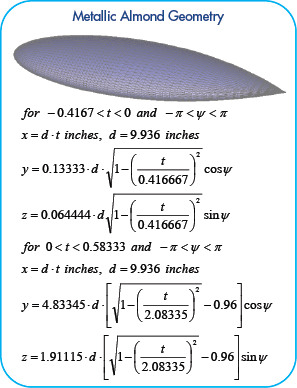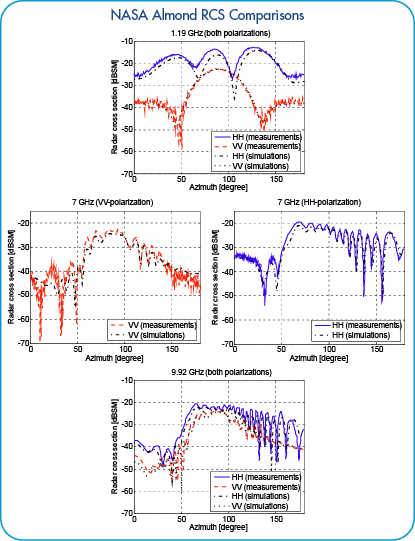Metallic Simple Ogive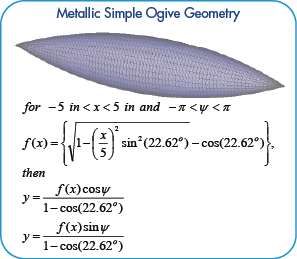The metallic ogive geometry and analytical expressions used for shape generation are shown below.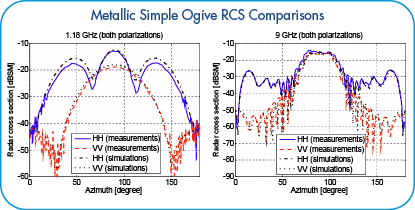The RCS for both horizontal and vertical polarization is plotted in dBSM as a function of the azimuthal angle.

Metallic Double Ogive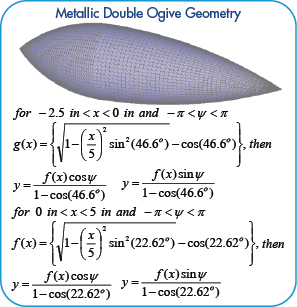The double ogive consists of two different-size half ogives. The metallic ogive geometry and analytical expressions used for shape generation are shown below.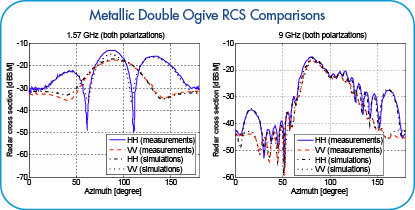The RCS for both horizontal and vertical polarization is plotted in dBSM as a function of the azimuthal angle.

Metallic Cone-sphere

The metallic cone-sphere geometry and analytical expressions used for shape generation are shown below.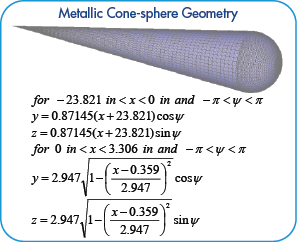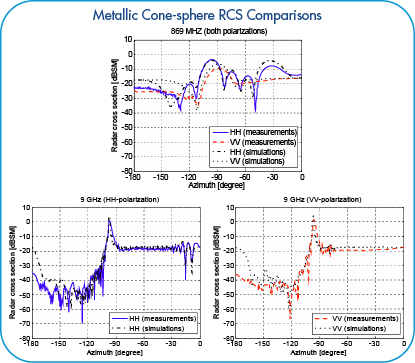The RCS for both horizontal and vertical polarization is plotted in dBSM as a function of the azimuthal angle.

Metallic Cone-sphere with Gap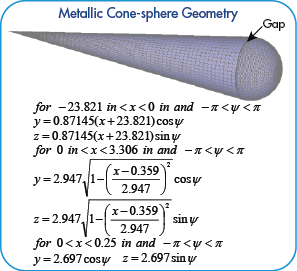The metallic cone-sphere geometry with gap and analytical expressions used for shape generation are shown below.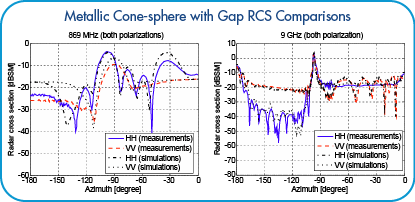The RCS for both horizontal and vertical polarization is plotted in dBSM as a func-tion of the azimuthal angle.

﻿